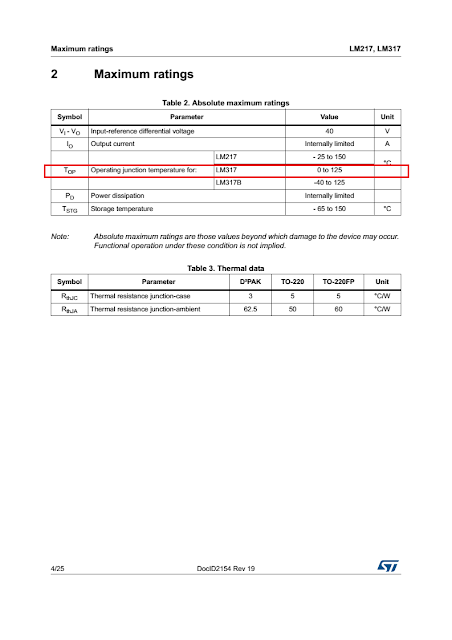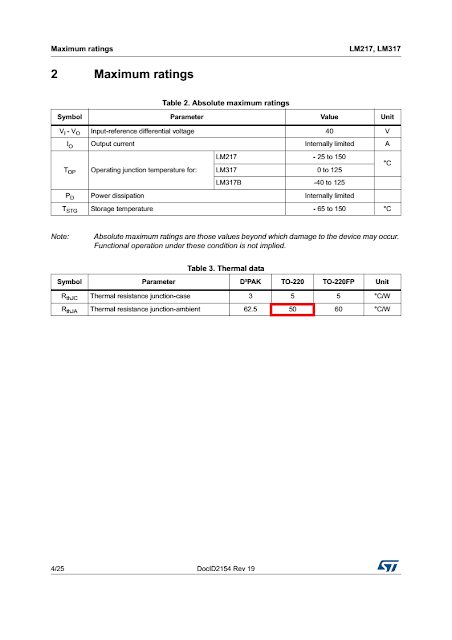## Why Do We Need A Heat Sink?

We need a heat sink to keep down the temperature of an electronic component. The electronic component could be the processor of a computer, a graphics card, a power transistor, a voltage regulator, or even a high power LED.

These things produce heat in their normal mode of operation. The heat is produced due to inherent electrical resistance of a material. The electrical resistance is an electrical property that impedes the flow of current through a material. Some electrical energy is lost when overcoming this resistance. This electrical energy gets converted to heat. If this heat is not taken away from the electronic component, the temperature of the component would rise leading to permanent failure of the component. A heat sink helps us in taking this heat away from the electronic component.

While there are heat sinks for electronics such as processors, graphics cards, and LEDs, we will focus solely on heat sinks for voltage regulators in this article.Some aluminum heat sinks of different thermal resistances, the first one from the right has LM7815 mounted on.

## What are Voltage Regulators?

Voltage regulators regulate voltage to a set value. There are AC voltage regulators as well as DC voltage regulators. AC voltage regulators regulate the mains voltage while DC voltage regulators regulate dc voltage and are used in power supplies for electronics such as desktop computers, laptop computers, uninterruptible power supplies etc.

DC voltage regulators come with a fixed or an adjustable output. Some dc voltage regulators with a fixed output voltage are

Fixed Voltage RegulatorsFixed Output Voltage
7805+5 V
7809+9 V
7812+12 V
7815+15 V

Some adjustable voltage regulators are LM317, LM338. These two are linear voltage regulators. DC voltage regulators can be further categorized as switching voltage regulators, positive voltage regulators, negative voltage regulators (output a negative voltage).

## When Should We Use A Heat Sink With A Voltage Regulator?

We should use a heat sink when the junction temperature, TJ of a voltage regulator is likely to exceed a maximum value. Inside the voltage regulator there is a small semiconductor die. The junction temperature is the temperature of this semiconductor die.Semiconductor die inside the case of a transistor

The absolute maximum junction temperature, TJ(max) of LM317, an adjustable voltage regulator, is 125°C.Absolute maximum junction temperature of LM317 by ST Microelectronics

LM317 Datasheet by ST Microelectronics

Find this value on page number 4 in Table 2. "Absolute maximum ratings"

## What causes an increase in junction temperature?

A linear voltage regulator such as LM317 dissipates some power in its normal mode of operation. This dissipated power appears in the form of heat, thus increasing the junction temperature.

The power dissipated by a voltage regulator such as LM317 can be given as

PD = ( VI - VO ) × IO ... (i)
where,
PD is power dissipated by LM317
VI is input voltage at the input pin of LM317 with respect to ground of the circuit
VO is output voltage at the output pin of LM317 with respect to ground of the circuit
IO is the output current or load

In the circuit of the mini UPS, when the output current is 1 A there are around 22 volts at the input of the LM317 and around 13 volts at the output. Thus, PD will be,

PD = ( VI - VO ) × IO
PD = ( 22 V - 13 V ) × 1 A
PD = 9 W

## What will be the junction temperature at this power dissipation?

To find the junction temperature at power dissipation of 9 W, consider the power dissipation as a function of TJ, TA, and RθJA

where,
TJ is junction temperature
TA is ambient temperature
RθJA is thermal resistance from junction to ambient

The equation (ii) is also mathematical form of Newton's law of cooling.

Thermal resistance is analogous to electrical resistance. The unit of electrical resistance is Ω while the unit of thermal resistance is °C/W.

The temperature at one side of a material, say, a wall, will be different than the temperature at the other side of the wall due to this thermal resistance just as electric potential at one side of a resistor is different than the electric potential at other side of the resistor (when measured with respect to a common reference).

Thus, electric potential difference is analogous to temperature difference. The electric potential difference causes current flow and temperature difference causes heat flow.

Let us dig into this deeper and try to derive equation (ii).

The heat has to flow from semiconductor die of voltage regulator to outside ambient air. First the heat travels from the junction to the case of the voltage regulator. Then from the case of the voltage regulator the heat escapes to the environment.

If we attach a heat sink with the voltage regulator, then the heat first travels from the case of the voltage regulator to the heat sink and then from the heat sink to the environment.

Accordingly, we get these thermal resistances,

RθJC = voltage regulator thermal resistance (from junction to case)
RθCH = interface material thermal resistance (from case to heat sink)
RθHA = heat sink thermal resistance (heat sink to ambient)

RθCH is the thermal resistance of the interface material that sits between voltage regulator and the heat sink. This interface material is commonly thermal grease, heat sink compound, heat sink paste etc. The heat sink compound fills in the air gaps that exist due to roughness of two surfaces. The air being thermal insulator can decrease the flow of heat and thus prompting the use of thermal grease or heat sink compound.

An equivalent electrical circuit can now be drawn to further help visualize the problem.Equivalent Electrical Circuit

where,
Q is rate of flow of heat and is analogous to electrical current
TJ is junction temperature and is analogous to electric potential
TC is case temperature and is analogous to electric potential
TH is the temperature of heat sink and is analogous to electric potential
TA is ambient temperature and is analogous to electric potential

Solving Ohm's law for current, we have

Using thermal to electrical analogy, the equivalent electric circuit shown above, and the Ohm's law we can find the rate of heat flow or electric current, (electric current is analogous to rate of flow of heat, electric potential difference is analogous to temperature difference, electrical resistance is analogous to thermal resistance)

Since the unit of measurement for heat flow is same as power (watts), thus

Summing the thermal resistances just as we sum electrical resistances, we have

which is same as eq. (ii).

Let us return to the question of finding junction temperature at power dissipation of 9 W when heat sink is not used. RθJA for LM317 (TO-220 package) is 50 °C/W.Thermal resistance from juction to ambient LM317 ST Microelectronics

Solving equation (ii) for TJ when TA = 25°C, PD = 9 W, RθJA = 50 °C/W.

That's much greater than absolute maximum junction temperature of 125°C. Thus, a heat sink should be used.

Which heat sink to use? The answer lies in finding the thermal resistance of the heat sink, RθHA, that would not allow the junction temperature to increase above absolute maximum temperature of 125°C.

Solving equation (iii) for RθHA when RθJC = 5 °C/W, RθCH = 1 °C/W, TJ = 125°C, TA = 25°C, PD = 9 W,

The thermal resistance of the selected heat sink should be lower than 5.11 °C/W.

RθCH is the thermal resistance of the interface material that sits between voltage regulator and the heat sink. When this is not known, we can assume that it is 1 °C/W.

RθJC is the thermal resistance from junction to case of the voltage regulator. It is different for different voltage regulators and can be found from the datasheet provided by the manufacturer. It is typically 5 °C/W.

We see that the thermal resistance from junction to ambient (using no heat sink) is 50 °C/W. This means that for each 1 W of power dissipation the junction temperature is 50°C above ambient temperature.

Now when the heat sink is used, the thermal resistance from junction to ambient becomes,

RθJC + RθCH + RθHA = 5 °C/W + 1 °C/W + 5.11 °C/W
= 11.11 °C/W

Thus, for each 1 W of power dissipation the junction temperature is only 11.11°C above ambient, compared to 50°C above ambient for the voltage regulator operating directly into free air.

The heat sink provides a low thermal resistance path between case and ambient and effectively increases the surface area through which the heat escapes into the atmosphere. From junction to case, and then from case to heat sink, the heat transfer is through conduction while from heat sink to ambient the heat transfer is through convection. This convection takes two forms

1. Natural Convection
2. Forced Convection

The forced convection involves the use of a fan that increases flow of air. The natural convection makes use of natural flow of air. The forced convection becomes useful when the space is limited and a big heat sink can not be accommodated in a small space. The process of finding the thermal resistance of the required heat sink in this tutorial is for a heat sink with natural convection. The process for forced convection is similar.

Throughout the tutorial I have tried to write as clearly as possible. Still, if you don't understand something and have questions, feel free to ask in the comments below.